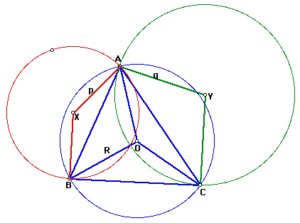# Circles & TrianglesLet ABC be a triangle with circumradius R (i.e., the radius of the circumcircle that has center O). Suppose that X and Y are circles such that both circles X and Y pass through the point A. Segment BC is tangent to circle X at B and Segment BC is tangent to circle Y at C. Let p be the radius of circle X and let q be the radius of circle Y. What is the relationship between R, p and q?